Longest common substring

Not to be confused with Longest common subsequence.

The longest common substring (LCS) problem is the problem of finding a string of maximum length which is simultaneously a substring of two or more given strings (usually two). For example, the longest common substring of atlas and elastic is las. This problem is much less applicable (in real life) than the more error-tolerant longest common subsequence problem, but it is theoretically interesting.

Discussion

We can observe immediately that this problem is solvable in polynomial time, because a string of length$n$ has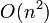$O(n^2)$ substrings, allowing us to simply list all substrings of each string and then find the longest entry(ies) common to both strings. This, however, gives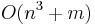$O(n^3+m)$ time (assuming we have two strings of lengths$n$ and$m$ and we use an efficient string search algorithm). In addressing the question of whether it is possible to do better, we at first consider three variations on the problem:

• Return all LCSes. There may only be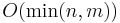$O(\min(n,m))$ of these, where$m$ is the length of the first string and$n$ is the length of the second. This is because there are only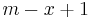$m-x+1$ substrings of any given length$x$ in the first string, and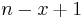$n-x+1$ in the second string.
• Return any LCS.
• Return only the length of an LCS.

We shall see that all three variations can be solved in linear time, no matter how many strings are given in input.

Dynamic programming solution

Dynamic programming admits a solution with time and space polynomial in the lengths of the strings involved but exponential in the number of strings. Nevertheless, it is the recommended method for two strings of length at most a few thousand, because of its simplicity. The reasoning is along the same lines as that used to solve the longest common subsequence problem. Let the two strings be denoted$S$ and$T$, where$S$ has characters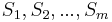$S_1, S_2, ..., S_m$ and$T$ has characters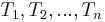$T_1, T_2, ..., T_n$. Define$f(i,j)$ for each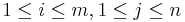$1 \leq i \leq m, 1 \leq j \leq n$ to be the length of the longest common substring ending at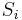$S_i$ and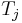$T_j$. Then:

1. Define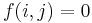$f(i,j) = 0$ if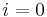$i = 0$ or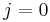$j = 0$.
2. Observe that if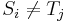$S_i \neq T_j$, then$f(i,j) = 0$.
3. If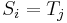$S_i = T_j$, then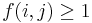$f(i,j) \geq 1$. In particular, if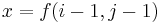$x = f(i-1,j-1)$, that is, there is a common substring of length$x$ ending at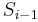$S_{i-1}$ and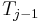$T_{j-1}$ (but no longer), then appending$S_i$ and$T_j$ gives a common substring of length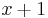$x+1$ ending at$S_i$ and$T_j$. Thus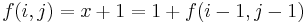$f(i,j) = x+1 = 1 + f(i-1,j-1)$.
4. Clearly, the length of the LCS overall is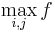$\max_{i,j} f$. Each pair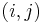$(i,j)$ for which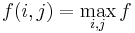$f(i,j) = \max_{i,j} f$ corresponds to a longest common substring ending at$S_i$ and$T_j$.

Thus:

input S, T
m ← length S
n ← length T
result ← 0
for i ∈ [0..m]
for j ∈ [0..n]
if i=0 or j=0 or S[i] ≠ T[j]
a[i][j] ← 0
else
a[i][j] ← 1 + a[i-1][j-1]
result ← max(result,a[i][j])
print "Longest common substring has length " + result

For three or more strings, the code is very similar, but each extra string introduces a new level of loop nesting. The time and memory used are proportional to the product of the lengths of all input strings, giving$O(mn)$ for the two-string case.

More efficient solution

The fastest solutions are obtained using suffix data structures.

Suffix tree

A generalized suffix tree of the strings involved can be constructed in linear time, assuming an integer alphabet. The LCS is then the one corresponding to the deepest node that contains labels for all input strings. If there are multiple longest common substrings, each one will correspond to a maximally deep node.

Proof: Clearly any node that contains labels from all input strings corresponds to a common substring of all input strings, and the length of this substring is given by the depth of the node. It only remains to show that the LCS always occurs at a node (and not along any of the edges). Suppose the LCS does occur in the middle of an edge. Then follow the edge down to the nearest node. If this node contains labels from all input strings, then it corresponds to a longer common substring, a contradiction. If it does not, then the edge should have split further up, yet it has not, also a contradiction.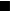$_\blacksquare$

Suffix array

Solution using a generalized suffix array is more complicated, but uses less space and may also be faster. The suffix array itself may be constructed in linear time, again assuming an integer alphabet. The longest common prefix array (LCP array) can also be constructed in linear time. Observe that if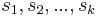$s_1, s_2, ..., s_k$ are consecutive suffixes in the suffix array, then the lengths of the longest common prefixes of the pairs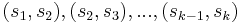$(s_1, s_2), (s_2, s_3), ..., (s_{k-1},s_k)$ are consecutive entries in the LCP array and the minimum value in this range is the length of the LCP of all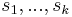$s_1, ..., s_k$ taken together. Observe further that to each common substring there corresponds such a set of suffixes containing at least one suffix from each input string, and the common substring is a common prefix of all the selected suffixes. (In particular, as a substring is always a prefix of a suffix, there must be a suffix from each input string which has the common substring as a prefix. Choose one such suffix from each input string; then take for the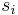$s_i$'s these suffixes and all the ones between them.)

Now, move a sliding window from left to right through the suffix array; the left edge should merely iterate from left to right, and the right edge should always move just far right enough to ensure that the window contains at least one suffix from each input string. (Observe that the right edge does, indeed, always stay stationary or move to the right; it never moves to the left.) The sliding window in the suffix array corresponds to a sliding window in the LCP array. For each position of the window in the LCP array let us find the minimum value of the LCP; this is then the length of the longest common prefix of the strings currently in the window in the suffix array. The maximum of these minimum values gives the LCS, since each window is only as large as it has to be to include all strings (and expanding a window can never increase the LCP length). This sliding range minimum query can be solved in linear time using a deque.